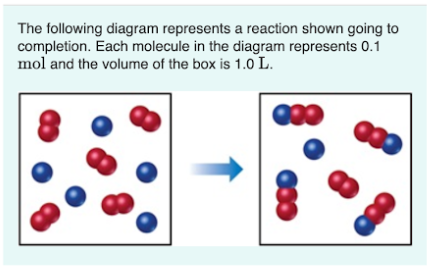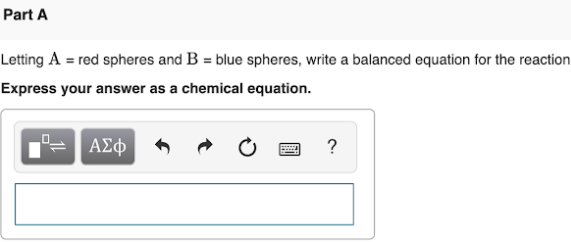# The following diagram represents a reaction shown going to completion. Each molecule in the diagram represents 0.1 mol and the volume of the box is 1.0 L. Letting A = red spheres and B = blue spheres, write a balanced equation for the reaction. Express your answer as a chemical equation.